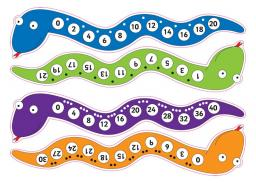# Equation 3364

Create a number snake from the equation and solve:
2x - 5 = 7
4x + 1/3 = 7
3 (x-2) +4 = 7

a =  6
b =  1.6667
c =  3

### Step-by-step explanation:

2a - 5 = 7

2·a - 5 = 7

2a = 12

a = 12/2 = 6

a = 6

Our simple equation calculator calculates it.

4b+1/3 = 7

4·b+1/3 = 7

12b = 20

b = 20/12 = 1.66666667

b = 5/3 ≈ 1.666667

Our simple equation calculator calculates it.

3(c-2)+4 = 7

3·(c-2)+4 = 7

3c = 9

c = 9/3 = 3

c = 3

Our simple equation calculator calculates it.Did you find an error or inaccuracy? Feel free to write us. Thank you!

Tips for related online calculators
Do you have a linear equation or system of equations and looking for its solution? Or do you have a quadratic equation?